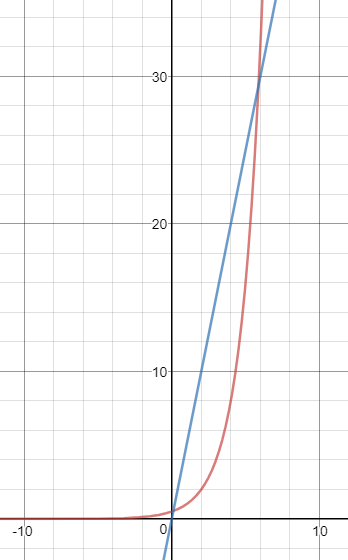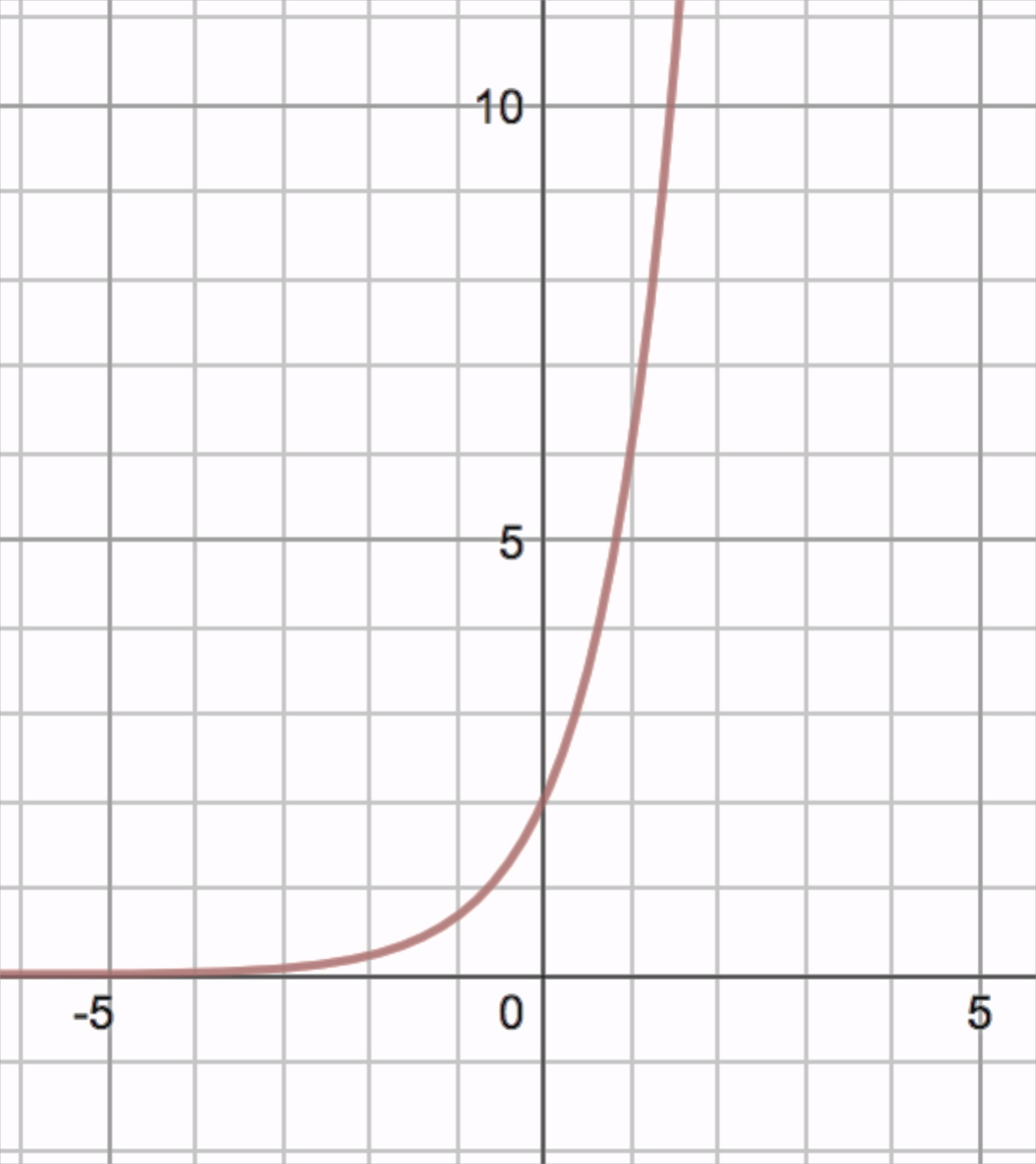# Exponents and Exponential Functions

## Objective

Compare rates of change in linear and exponential functions shown as equations, graphs, and situations.

## Common Core Standards

### Core Standards

?

• F.IF.C.9 — Compare properties of two functions each represented in a different way (algebraically, graphically, numerically in tables, or by verbal descriptions). For example, given a graph of one quadratic function and an algebraic expression for another, say which has the larger maximum.

• F.LE.A.1 — Distinguish between situations that can be modeled with linear functions and with exponential functions.

• F.LE.A.3 — Observe using graphs and tables that a quantity increasing exponentially eventually exceeds a quantity increasing linearly, quadratically, or (more generally) as a polynomial function.

• A.SSE.A.1 — Interpret expressions that represent a quantity in terms of its context Modeling is best interpreted not as a collection of isolated topics but in relation to other standards. Making mathematical models is a Standard for Mathematical Practice, and specific modeling standards appear throughout the high school standards indicated by a star symbol (★). The star symbol sometimes appears on the heading for a group of standards; in that case, it should be understood to apply to all standards in that group.

?

• 8.F.A.2

• 8.F.B.4

## Criteria for Success

?

1. Understand that linear functions have a constant rate of change and exponential functions have an increasing or decreasing rate of change.
2. Identify whether functions are linear or exponential in graphs, tables, equations, and situations.
3. Compare linear and exponential functions, identifying where the rates of change of exponential functions are greater than or less than linear functions.
4. Understand that an increasing exponential function will eventually exceed an increasing linear function.

## Tips for Teachers

?

This lesson focuses on understanding and making connections across situations, graphs, and equations of linear and exponential functions. Students are not asked to generate equations, graphs, or contexts; they will work on those skills and concepts in the lessons that follow.

## Anchor Problems

?

### Problem 1

Complete "Avi and Benita's Repair Shop" by Desmos.

#### Guiding Questions

Create a free account or sign in to access the Guiding Questions for this Anchor Problem.

#### References

Avi and Benita's Repair Shop by Desmos is made available by Desmos. Copyright © 2017 Desmos, Inc. Accessed May 17, 2018, 12:43 p.m..

### Problem 2

Two equations are shown below.

A:   ${y=0.01(2^x)}$

B:   ${y=100x}$

1. Which equation represents Avi’s payment rule and which equation represents Benita’s payment rule?
2. Explain what each part of each equation means in context of the situation.
3. Describe, using features from the equations, how each function grows over time.

#### Guiding Questions

Create a free account or sign in to access the Guiding Questions for this Anchor Problem.

### Problem 3

Two equations and their graphs are shown.Equation 1:  ${y=5x}$

Equation 2:  ${y=0.5(2^x)}$

a)  Label each graph with the appropriate equation.

b)  Describe the change in $y$ in each function as $x$ increases by $1$

c)  Describe the behavior of the exponential graph over time, as compared to the linear graph.

d)  Over approximately what interval is the linear function greater than the exponential function?

#### Guiding Questions

Create a free account or sign in to access the Guiding Questions for this Anchor Problem.

## Problem Set

?

The following resources include problems and activities aligned to the objective of the lesson that can be used to create your own problem set.

• Include examples where students are given different representations of linear and exponential functions (tables, graphs, equations), and must match them together

## Target Task

?

Use the function shown below to answer the questions that follow.a.  Which equation matches the graph?

i.   ${y=3(2^x )}$

ii.   ${y=2(3^x )}$

iii.   ${y=4x+2}$

iv.   ${y=2x+4}$

b.  Explain why you chose that equation in part (a).

c.  What is the rate of change of this function?

d.  Suppose the graph of ${y=150x}$ was added to the graph above. Which function is greater over the domain interval ${1<x<5}$? Will this function always be greater over all values of the domain? Explain your reasoning.